Question

Suppose that a researcher, using data on class size (CS) and average test scores from 100...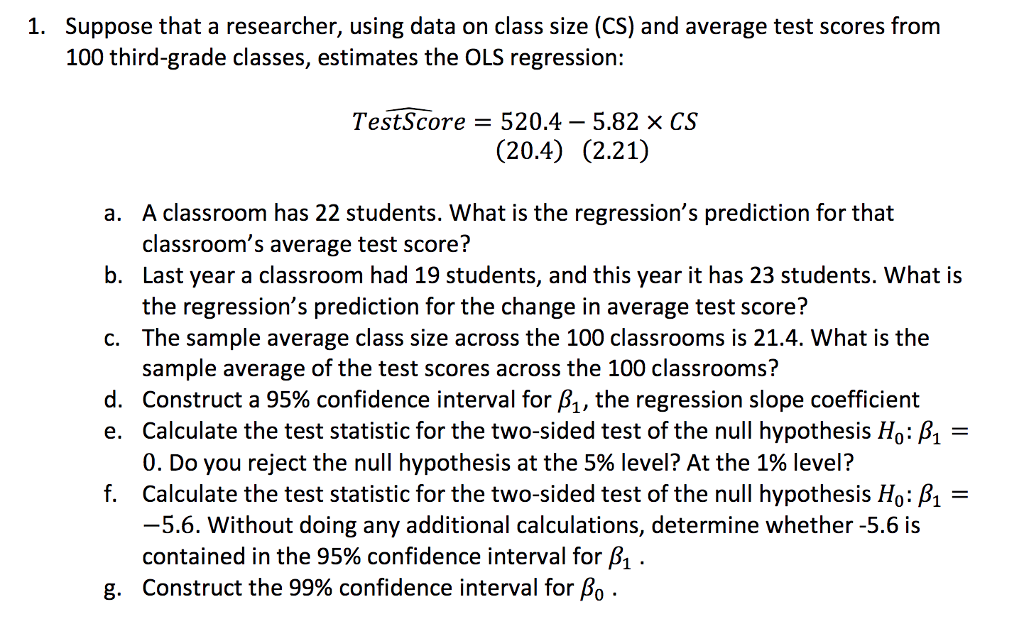Suppose that a researcher, using data on class size (CS) and average test scores from 100 third-grade classes, estimates the OLS regression: 1. Test-Score = 520.4-5.82 × CS (20.4) (2.21) A classroom has 22 students. What is the regression's prediction for that classroom's average test score? Last year a classroom had 19 students, and this year it has 23 students. What is the regression's prediction for the change in average test score? The sample average class size across the 100 classrooms is 21.4. What is the sample average of the test scores across the 100 classrooms? Construct a 95% confidence interval for A, the regression slope coefficient Calculate the test statistic for the two-sided test of the null hypothesis Ho: A- 0. Do you reject the null hypothesis at the 5% level? At the 1% level? Calculate the test statistic for the two-sided test of the null hypothesis Ho: -5.6. Without doing any additional calculations, determine whether -5.6 is contained in the 95% confidence interval for A . Construct the 99% confidence interval for Ao . a. b. c. d. e. f. g.

a)
y^= 520.4 - 5.82 * 22
= 392.36

b)
change in average test score = -5.82 ( 23 - 19)
= -23.28

it will reduce by 23.28 points

c)
y^= 520.4 - 5.82 * 21.4
= 395.852

d)

df =n-2 = 98
t = 1.9845

(-5.82 - 1.9845 * 2.21 , -5.82 + 1.9845 * 2.21 )
= ( -10.2057 , -1.4343 )

e)
since 0 is not present in 95% confidence interval
we reject the null at 5 % level

I have solved first 4 parts by HomeworkLib policy

Post e) to g) parts again

Earn Coins

Coins can be redeemed for fabulous gifts.

Similar Homework Help Questions
• PLEASE JUST PARTS F) AND G) Suppose that a researcher, using data on class size (CS)...PLEASE JUST PARTS F) AND G) Suppose that a researcher, using data on class size (CS) and average test scores from 100 third-grade classes, estimates the OLS regression: 1. Test-Score = 520.4-5.82 × CS (20.4) (2.21) A classroom has 22 students. What is the regression's prediction for that classroom's average test score? Last year a classroom had 19 students, and this year it has 23 students. What is the regression's prediction for the change in average test score? The sample...

• 5.1.) Suppose that a researcher, using data on class size (CS) and average test scores from 100 third-grade classes, es...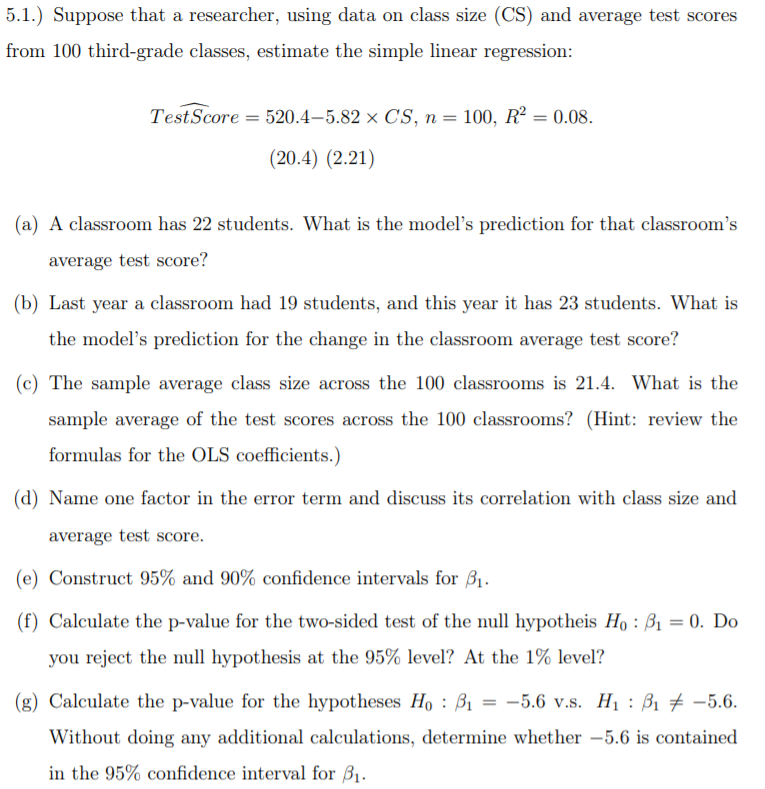5.1.) Suppose that a researcher, using data on class size (CS) and average test scores from 100 third-grade classes, estimate the simple linear regression: Test Score = 520.4-5.82 x CS, n= 100, R2 = 0.08. (20.4) (2.21) (a) A classroom has 22 students. What is the model's prediction for that classroom's average test score? (b) Last year a classroom had 19 students, and this year it has 23 students. What is the model's prediction for the change in the classroom...

• (h) Construct a 99% confidence interval for β0. 5.1.) Suppose that a researcher, using data on class size (CS) and aver...(h) Construct a 99% confidence interval for β0. 5.1.) Suppose that a researcher, using data on class size (CS) and average test scores from 100 third-grade classes, estimate the simple linear regression: Test Score = 520.4-5.82 x CS, n= 100, R2 = 0.08. (20.4) (2.21) (a) A classroom has 22 students. What is the model's prediction for that classroom's average test score? (b) Last year a classroom had 19 students, and this year it has 23 students. What is the...

• 1. Suppose that a researcher, using data on class size (CS) and average test scores from...

1. Suppose that a researcher, using data on class size (CS) and average test scores from 100 third-grade classes, estimates the OLS regression, T esdtScore = 520:4 5:82 CS A classroom has 22 students. Whatís the regressionís prediction for that classroomís average test score? Last year a classroom had 19 students, and this year it has 23 students. What is the regressionís prediction for the change in the classroom average test score? The sample average class size across the 100...

• Suppose that a researcher, using data on class size (CS) and average test scores from 103...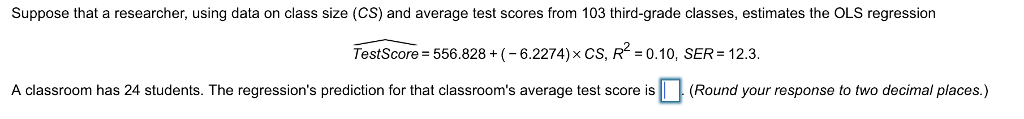Suppose that a researcher, using data on class size (CS) and average test scores from 103 third-grade classes, estimates the OLS regression TestScore = 556.828 + (-6.2274) x CS, R2-0.10, SER-123. A classroom has 24 students. The regression's prediction for that classroom's average test score is(Round your response to two decimal places.)

• Suppose that a researcher, using data on class size (CS) and average test scores from 105...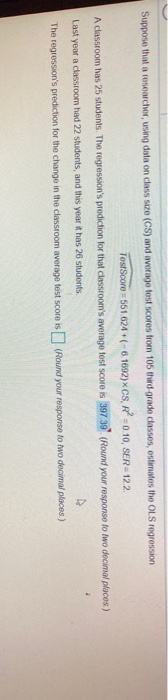Suppose that a researcher, using data on class size (CS) and average test scores from 105 third grade classes, estimates the OLSrogression TestScore 551.624 +(-6.1002)XCS, R = 0.10, SER=122. A classroom has 25 students. The regression's prediction for the classroom's average test score is 397.39 (Round your response to two decimal places) Last you a classroom had 22 students, and this year it has 26 students A The regression's prediction for the change in the classroom average test score is...

• From Stock & Watson (2019) Exercise 5.1: A researcher, using data on class size (CS) and...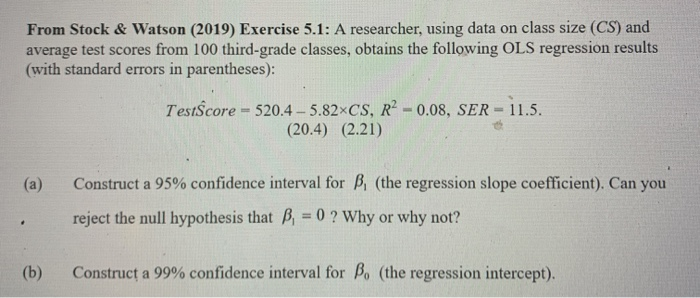From Stock & Watson (2019) Exercise 5.1: A researcher, using data on class size (CS) and average test scores from 100 third-grade classes, obtains the following OLS regression results (with standard errors in parentheses): TestScore = 520.4-5.82xCS, R2 -0.08, SER - 11.5. (20.4) (2.21) (a) Construct a 95% confidence interval for B, (the regression slope coefficient). Can you reject the null hypothesis that B, = 0 ? Why or why not? (b) Construct a 99% confidence interval for B. (the...

• 1. (Based on Stock & Watson “Introduction to Econometrics” 6th ed., Exercise 4.1 and 5.1.) Suppose...

1. (Based on Stock & Watson “Introduction to Econometrics” 6th ed., Exercise 4.1 and 5.1.) Suppose that a researcher, using data on class size (CS) and average test scores from 100 third-grade classes, estimate the simple linear regression: TestScore d = 520.4−5.82 × CS, n = 100, R^2 = 0.08. (20.4) (2.21) (d) Name one factor in the error term and discuss its correlation with class size and average test score. (e) Construct 95% and 90% confidence intervals for β1....

• 0/1 pts Incorrect Question1 Binary variables are generally used to control for outliers in your sample...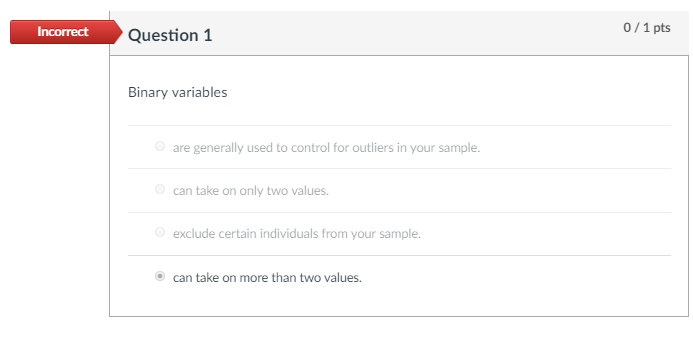0/1 pts Incorrect Question1 Binary variables are generally used to control for outliers in your sample can take on only two values exclude certain individuals from your sample. O can take on more than two values 0/1 pts Question 5 Suppose that a researcher, using data on class size (CS)and average test scores from 103 third-grade classes, estimates the OLS regression Testscore-556.828 - 6.2274CS, R2-.09, SER 12.3. (a) A classroom has 20 students. The regression's prediction for that classroom's average...

• (4)Five hundred students from a local high school took a college entrance examination. Historical data from the school record show that the standard deviation of test scores is 40. A random sampl...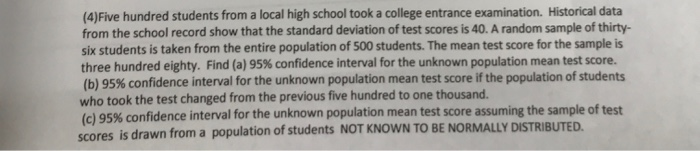(4)Five hundred students from a local high school took a college entrance examination. Historical data from the school record show that the standard deviation of test scores is 40. A random sample of thirty- six students is taken from the entire population of 500 students. The mean test score for the sample is three hundred eighty. Find (a) 95% confidence interval for the unknown population mean test score. (b) 95% confidence interval for the unknown population mean test score if...